# Canny检测算法与实现

## 1、原理

Canny 边缘检测算法是John F. Canny 于1986年开发出来的一个多级边缘检测算法，也被很多人认为是边缘检测的 最优算法, 最优边缘检测的三个主要评价标准是:

Canny算子求边缘点具体算法步骤如下：

1. 用高斯滤波器平滑图像．

2. 用一阶偏导有限差分计算梯度幅值和方向.

3. 对梯度幅值进行非极大值抑制.

4. 用双阈值算法检测和连接边缘．

## 2、实现步骤

2.1、消除噪声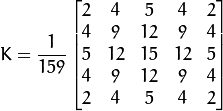2.2、计算梯度幅值和方向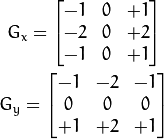在vs中可以看到sobel像素值和形状：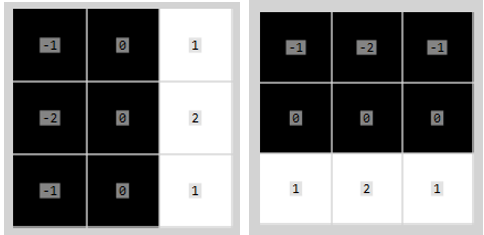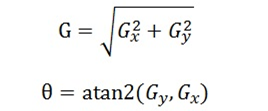2.3、非极大值抑制

1）  0：左边 和 右边

2） 45：右上 和 左下

3） 90：上边 和 下边

4）135：左上 和 右下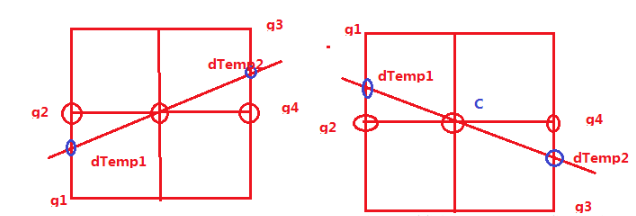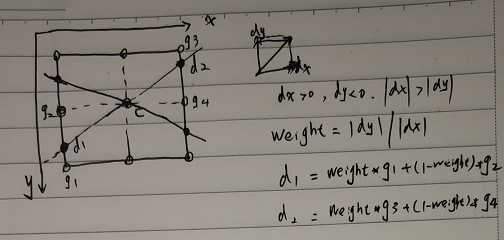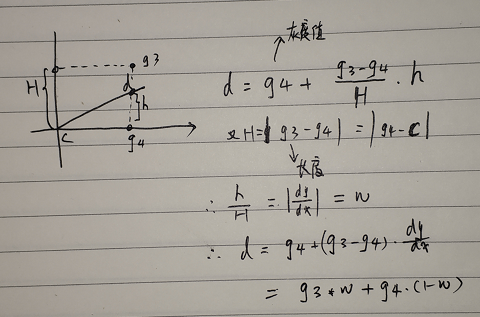Matlab使用非常有技巧的方式来计算方向，如下不仅做了dx、dy的大小判断还做了方向的判定。

`witch direction      case 1          idx = find((iy<=0 & ix>-iy)  | (iy>=0 & ix<-iy));      case 2          idx = find((ix>0 & -iy>=ix)  | (ix<0 & -iy<=ix));      case 3          idx = find((ix<=0 & ix>iy) | (ix>=0 & ix<iy));      case 4          idx = find((iy<0 & ix<=iy) | (iy>0 & ix>=iy));  end`

2.4、双阈值检测和区域连通

## 3、代码实现

3.1 计算梯度

`/*  * Sobel 梯度计算  */  Mat gradients(Mat &img, Mat &sobel)  {      int W = img.cols;      int H = img.rows;        Mat dx = Mat_<int>(img.size());      int border = (int)sobel.rows / 2;        for (int r = border; r < H - border; r++)      {          for (int c = border; c < W - border; c++)          {              float tmp = 0;              for (int i = -border; i <= border; i++) {                  for (int j = -border; j <= border; j++) {                      tmp += (int)img.data[(r + i)*W + c + j] * sobel.at<int>(i + border, j + border);                  }              }                dx.at<int>(r, c) = tmp;          }      }      return dx;  }`

3.2计算非极大值抑制（详细推导过程见参考文献文章）

`/*  fucntion: non-maximum suppression  input:  pMag:   pointer to Magnitude,  pGradX: gradient of x-direction  pGradY: gradient of y-direction  sz: size of pMag (width = size.cx, height = size.cy)  limit: limitation  output:  pNSRst: result of non-maximum suppression  */  void NonMaxSuppress(int *pMag, int * pGradX, int *pGradY, Size sz, int *pNSRst)  {      long x, y;      int nPos;      // the component of the gradient      int gx, gy;      // the temp varialbe      int g1, g2, g3, g4;      double weight;      double dTemp, dTemp1, dTemp2;      //设置图像边缘为不可能的分界点      for (x = 0; x < sz.width; x++)      {          pNSRst[x] = 0;          pNSRst[(sz.height - 1)*sz.width + x] = 0;      }      for (y = 0; y < sz.height; y++)      {          pNSRst[y*sz.width] = 0;          pNSRst[y*sz.width + sz.width - 1] = 0;      }        for (y = 1; y < sz.height - 1; y++)      {          for (x = 1; x < sz.width - 1; x++)          {              nPos = y * sz.width + x;              // if pMag[nPos]==0, then nPos is not the edge point              if (pMag[nPos] == 0)              {                  pNSRst[nPos] = 0;              }              else              {                  // the gradient of current point                  dTemp = pMag[nPos];                  // x,y 方向导数                  gx = pGradX[nPos];                  gy = pGradY[nPos];                  //如果方向导数y分量比x分量大，说明导数方向趋向于y分量                  if (abs(gy) > abs(gx))                  {                      // calculate the factor of interplation                      weight = fabs(gx) / fabs(gy);                      g2 = pMag[nPos - sz.width];  // 上一行                      g4 = pMag[nPos + sz.width];  // 下一行                      //如果x,y两个方向导数的符号相同                      //C 为当前像素，与g1-g4 的位置关系为：                      //g1 g2                      //   C                      //   g4 g3                      if (gx*gy > 0)                      {                          g1 = pMag[nPos - sz.width - 1];                          g3 = pMag[nPos + sz.width + 1];                      }                      //如果x,y两个方向的方向导数方向相反                      //C是当前像素，与g1-g4的关系为：                      //    g2 g1                      //    C                      // g3 g4                      else                      {                          g1 = pMag[nPos - sz.width + 1];                          g3 = pMag[nPos + sz.width - 1];                      }                  }                  else                  {                      //插值比例                      weight = fabs(gy) / fabs(gx);                      g2 = pMag[nPos + 1]; //后一列                      g4 = pMag[nPos - 1];    // 前一列                      //如果x,y两个方向的方向导数符号相同                      //当前像素C与 g1-g4的关系为                      // g3                      // g4 C g2                      //       g1                      if (gx * gy > 0)                      {                          g1 = pMag[nPos + sz.width + 1];                          g3 = pMag[nPos - sz.width - 1];                      }                        //如果x,y两个方向导数的方向相反                      // C与g1-g4的关系为                      // g1                      // g4 C g2                      //      g3                      else                      {                          g1 = pMag[nPos - sz.width + 1];                          g3 = pMag[nPos + sz.width - 1];                      }                  }                    dTemp1 = weight * g1 + (1 - weight)*g2;                  dTemp2 = weight * g3 + (1 - weight)*g4;                  if(dTemp )                  //当前像素的梯度是局部的最大值                  //该点可能是边界点                  if (dTemp >= dTemp1 && dTemp >= dTemp2)                  {                      pNSRst[nPos] = dTemp;                  }                  else                  {                      //不可能是边界点                      pNSRst[nPos] = 0;                  }              }          }      }  }`

3.3双阈值检测和边缘连接

`void duble_threshold(Mat &pMag, Mat &pThreadImg, float threshold)  {      double maxv;      int * img_ptr = pMag.ptr<int>(0);      uchar * dst_ptr = pThreadImg.ptr<uchar>(0);      minMaxLoc(pMag, 0, &maxv, 0, 0);      cout << "max" << maxv << endl;        int TL = 0.333 * threshold *maxv; // 1/3 of TH      int TH = threshold *maxv;      int w = pMag.cols;      int h = pMag.rows;        for (int r = 1; r < pMag.rows; r++)      {          for (int c = 1; c < pMag.cols; c++)          {              int tmp = img_ptr[r*w + c];              if (tmp < TL) {                  dst_ptr[r*w + c] = 0;              }              else if (tmp >= TH) {                  dst_ptr[r*w + c] = 255;              }              else {                  bool connect = false;                  for(int i=-1; i<=1 && connect == false; i++)                      for (int j = -1; j <= 1 && connect == false; j++)                      {                          if (img_ptr[r + i, c + j] >= TH)                          {                              dst_ptr[r*w + c] = 255;                              connect = true;                              break;                          }                          else  dst_ptr[r*w + c] = 0;                      }              }          }      }  }`

## 4、测试结论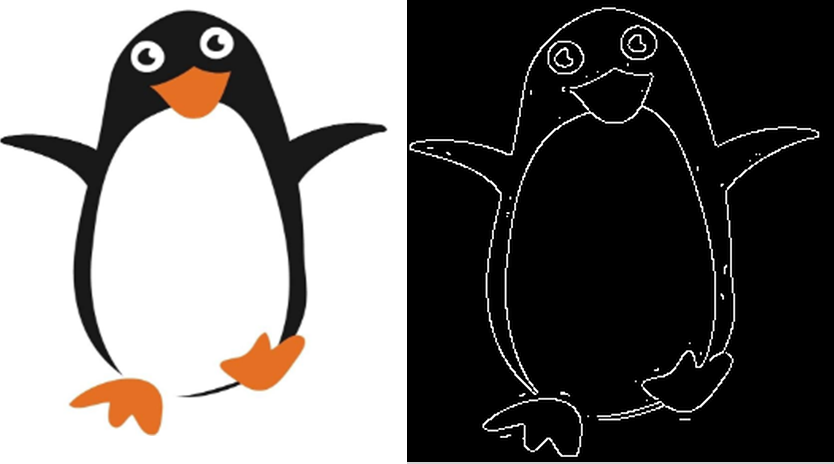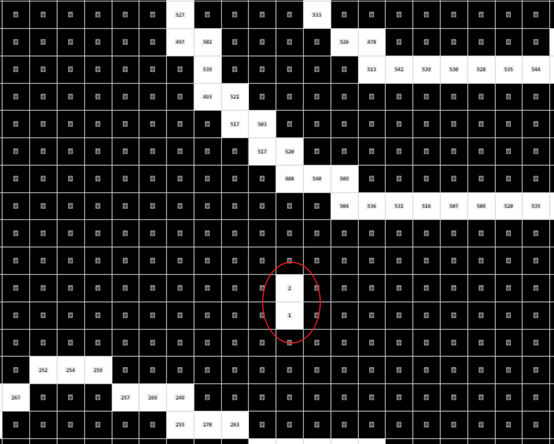使用双阈值限定后如下图，内部点消失了。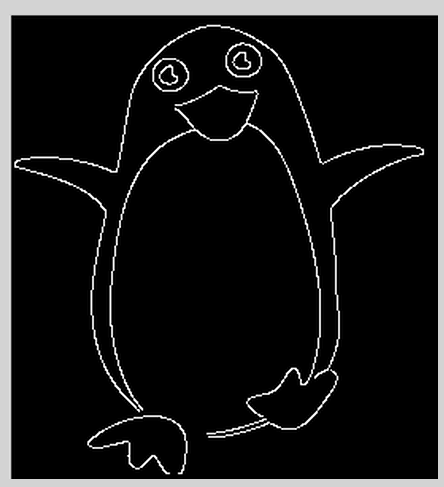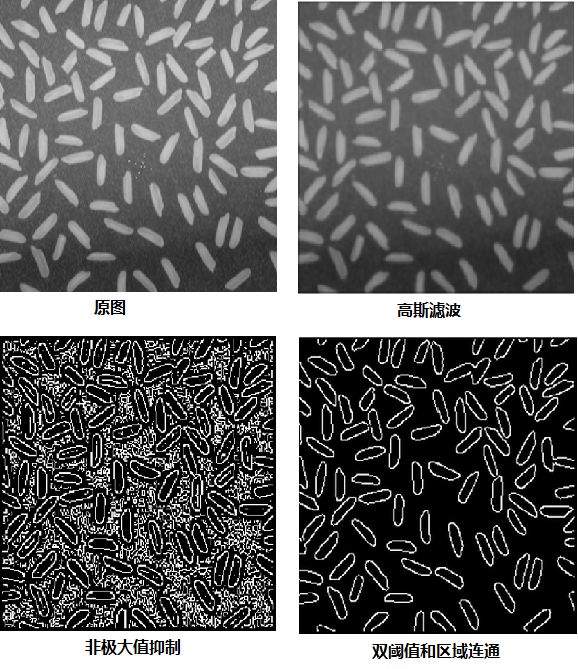##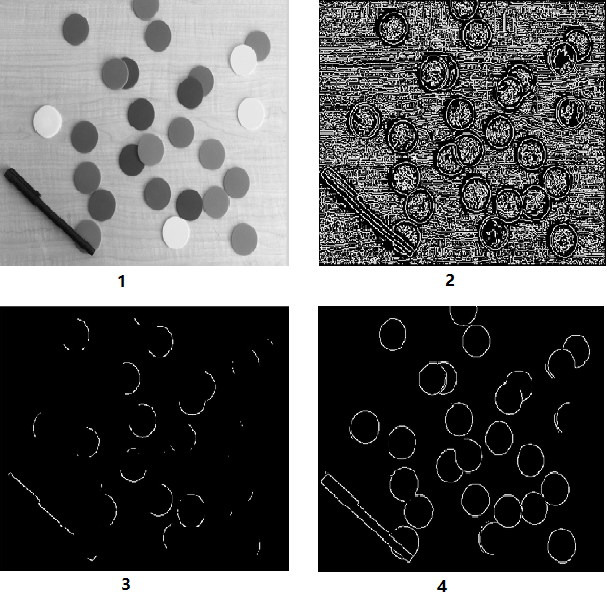5、参考文献

1、《数字图像处理与机器视觉》，第二版。 张铮、徐超、任淑霞、韩海玲等编著。

2、Canny 边缘检测

http://www.opencv.org.cn/opencvdoc/2.3.2/html/doc/tutorials/imgproc/imgtrans/canny_detector/canny_detector.html

3、Sobel算子的数学基础

http://blog.sciencenet.cn/blog-425437-1139187.html

4、Canny边缘检测

https://www.cnblogs.com/mmmmc/p/10524640.html

5、Canny算子中的非极大值抑制（Non-Maximum Suppression）分析

https://blog.csdn.net/kezunhai/article/details/11620357

6、一种改进非极大值抑制的Canny边缘检测算法

https://www.doc88.com/p-5174766661571.html